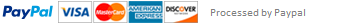### Python Assignment 3 - Solving Mathematical Problems

This assignment will give you experience on the use of:

1. Integers and floats

2. Mathematical operations

3. The float(), int(), round(), print(), and input()functions

4. Importing a Python module

The goal of this project is to gain experience with mathematical manipulation of numeric values. You will calculate areas and volumes to determine materials required for a gardening project.  The basic design consists of prompting the user for information, receiving information, processing that information, and then displaying the results.

Background

Programs are good at performing routine mathematical calculations. By way of illustration, you will write a program to calculate the materials needed for an ornamental garden according to the design below. In this design, the blue areas represent flowerbeds and the yellow areas are filled with stone, mulch, or other fill material. The garden is a perfect square. The four outer flowerbeds are congruent semicircles and the central flowerbed is a perfect circle.

Your program should prompt the user for the following information:

1. The side length (in feet) of the finished garden.

2. The recommended spacing (in feet) between plants.

3. The depth (in feet) of the flowerbeds.

4. The depth (in feet) of the filled areas.

Next estimate the number of plants and the amount of fill and flowerbed soil needed. Finally, it should report the following quantities needed for the garden:

1. Number of plants for each type of flowerbed (semicircle and circle) and total number of plants for the garden.

2. Cubic yards of soil for each type of flowerbed (semicircle and circle) and total cubic yards of soil for the garden, rounded to one decimal place.

3. Total cubic yards of fill material for the garden, rounded to one decimal place.

Criteria

Deliverables

1. Code: proj02.py – your source code solution (remember to include the date, project number and comments in this file).  Be sure to use “proj02.py” for the file name.  Be sure to add comments to your code to help me understand it.

2. Pseudocode: pseudo02.doc – your pseudocode solution to the problem in a Microsoft Word file.  Be sure to use proper indentation and “program” in English (or your first language of choice with an English translation).

3. Flowchart: flow02-partX.jpg – your flowchart solution in an image file format (jpg, png, etc.)  Be sure to use the proper shapes for each type of statement in your program.  Each separate function created should have its own flow image (numbered as flow02-part1.jpg, flow02-part2.jpg, etc.).

Assignment Notes:

To clarify the problem specifications, a snapshot of interaction with the already written program is provided at the end of this document.

The formula for the area A of a circle is A = r2, where r denotes the radius. For this calculation, you should use the value of provided in the Python math module. After importing the math module, the name math.pi returns (a close approximation to) the value of . (See comments in example_numeric.py.)

To estimate the number of plants for a flowerbed, divide the area of the flowerbed by the area needed per plant (the square of the recommended distance between plants) and then truncate this result.  Be careful, the number of plants in a semicircle may not be exactly half of the plants in a full circle.

Assume that the user only inputs numbers, that is, you don’t need to do error checking for bad input. Remember to convert fill to cubic yards.

Here is an outline of your program:

1. Prompt for input (use input)

2. Convert the input strings to numbers (use float)

3. Do some mathematical calculations using the input numbers to get results.

4. Print the results (use print)

Getting Started

1. Solve the problem using paper, pencil and calculator so you understand the problem before trying to program it.

2. Using Spyder, create a new program.

3. Save the project under the name: proj01.py

4. Run the program and track down any errors.

5. Now you enter a cycle of edit-run to incrementally develop your program.

6. Use Blackboard to submit the program.

Questions for you to consider

Please answer the following questions in the text box portion of the assignment submission page:

1. What happens when the user enters a letter instead of a number at the prompt?

2. What are two ways to get the program to crash (produce an error instead of printing output)?

3. Can you find inputs for which the number of plants required for the center circular flowerbed is not twice that required for a semi-circular bed?

Sample Interactions:

Calculate Garden requirements

-----------------------------

Enter length of side of garden (feet): 10

Enter spacing between plants (feet): 0.5

Enter depth of garden soil (feet): 0.8333

Enter depth of fill (feet): 0.8333

-----------------------------

Requirements

Plants for each semicircle garden: 39 Plants for the circle garden: 78

Total plants for garden: 234

Soil for each semicircle garden: 0.3 cubic yards

Soil for the circle garden: 0.6 cubic yards

Total soil for the garden: 1.8 cubic yards

Total fill for the garden: 1.3 cubic yards

This assignment has been answered 5 times in private sessions.